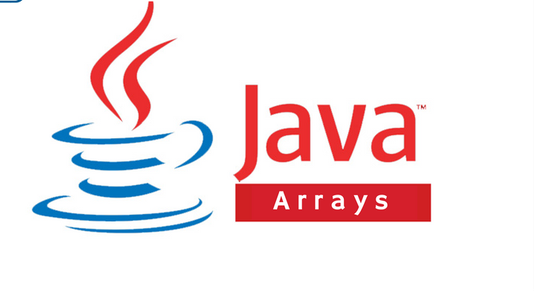# Number Class in JavaUsually in Java when we work with numbers, we will represent these numbers with any of the available primitive data type, such as int, byte, long, short, double, float.

### Example

``````int x = 50;
float y = 50.2f;
byte z = 4;``````

But, there could sometimes be special situations where we will need to use objects instead of the primitive data types.

Java provides a sleek way of achieving that with Wrapper Classes.

### What is a Wrapper Class?

Wrapper classes, which include Integer, Long, Short, Byte, Float and Double are actually subclasses of the Number Class.

the object of the wrapper class wraps whatever data type is passed into it, and when we convert primitive data types like this into objects, it is known as boxing.

The Wrapper Class is also converted back to the respective data type, and this is known as unboxing.

### Example of boxing

``````public class NumberClass{
public static void main(Strings args[]){
Double x = 4.5; //boxes double to Double object
x = x + 4.5; //unboxes Double to primitive double
System.out.println(x); //9
}
}``````

### Number Methods

There are more than 20 Number Methods that can be used in Java, but we’ll take examples of some of these methods and see how they are used:

### 1. equals():

This method determines whether the Number object that invokes the method is equal to the object that is passed as an argument.

``````public class Test{
public static void main(String args[]){
Integer x = 5;
Integer y = 10;
System.out.println(x.equals(y));
}
}``````

### 2. toString():

This returns a String object representing the value of the integer.

`` System.out.println(x.toString());``

### 3. round():

This method returns the closest long or int as given by the method’s return type. It takes a double of float.

``Math.round(45.5575565);``